Circle Passing through 3 points, Cyclic Quadrilateral and Related Theorems, Class 9, Mathematics

# Circle Passing through 3 points, Cyclic Quadrilateral and Related Theorems, Class 9, Mathematics - Extra Documents & Tests for Class 9

Circle through 3 Points

Prove that one and only one circle, passing through three non-collinear points.
Given :
Three non-collinear points A, B, C.
To prove : One and only one circle can be drawn, passing through A, B, and C.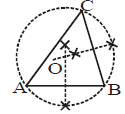Construction : Join AB and BC. Draw the perpendicular bisectors of AB O and BC. Let these perpendicular bisector intersect meeting at a point O.
Proof :

 STATEMENT REASON 1. O lies on the perpendicular bisector of AB⇒ OA = OB... (i) Every point on perpendicular bisector of a line segmentis equidistant from its end points i.e. A and B. 2. O lies on the perpendicular bisector of B⇒ OB = OC... (ii) Each point on perpendicular bisector of line segment isequidistant from its end points i.e. B and C. 3. OA = OB = OC⇒ O is equidistant from A, B and C⇒ Any circle drawn with centre O and radius OA will pass through B and C also. From (i) and (ii) 4. O is the only point equidistant from A, B andC. Perpendicular bisectors of AB and BC cut each other atpoint O only

Hence, one and only circle can be drawn through three non-collinear points A, B and C.

Cyclic Quadrilateral : If all the four vertices of a quadrilateral lie on a circle, then such a quadrilateral is called a cyclic quadrilateral.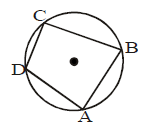If four points lie on a circle, they are said to be concyclic. We also say that quad. ABCD is inscribed in a circle with centre O.

Theorem-8. The opposite angles of a quadrilateral inscribed in a circle are supplementary.

OR

The sum of the opposite angles of a cyclic quadrilateral is 180°.
Given :
A quadrilateral ABCD inscribed in a circle with centre O.
To prove : ∠ADC + ∠ABC = 180° and ∠BAD + ∠BCD = 180°.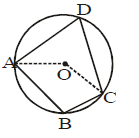Construction : Join OA and OC.
Proof :

 STATEMENT REASON 1. Arc ABC subtends ∠AOC at the centre and ∠ADC at a point D on the remaining part of the circle.∠AOC = 2 ∠ADC⇒∠ADC = 1/2∠AOC Angle at the centre is double the angle at any point on remaining part of the circle.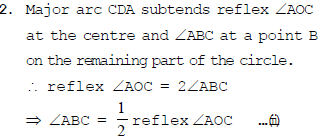Same as above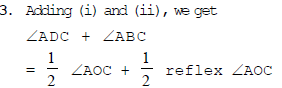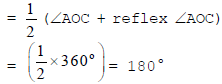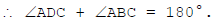(∠AOC+ reflex ∠AOC)=360°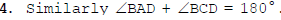= sum of the angles around a point O

Hence, the opposite angles of a cyclic quadrilateral are supplementary.

Converse of above Theorem : If a pair of opposite angles of a quadrilateral are supplementary, then the quadrilateral is cyclic.
Given :
A quadrilateral ABCD in which ∠B + ∠D = 180°.
To prove : ABCD is a cyclic quadrilateral.
Construction : If possible, let ABCD be not a cyclic
quadrilateral. Draw a circle passing through three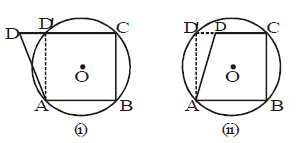non-collinear points A, B, C. Suppose this circle meets CD or CD produced at D', as shown in Fig. (i) and Fig. (ii) respectively. Join D'A.
Proof :

 STATEMENT REASON 1. ∠B + ∠D = 1800 Given. 2. ∠B + ∠D' = 1800 ABCD' is a cyclic quadrilateral and so its opposite ∠s are supplementary. 3. ∠B + ∠D = ∠B + ∠D'= ∠D = ∠D' From (i) and (ii). 4. But, this is not possible. A∴Our supposition is wrong.ABCD is a cyclic quadrilateral. An exterior angle of a Δ is never equal to its into opp. angle.

Hence proved

Theorem-9. The exterior angle of a cyclic quadrilateral is equal to the interior opposite angle.
Given :
A cyclic quadrilateral whose side AB is produced to a point E.
To prove : ∠CBE = ∠ADC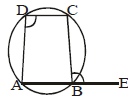Proof :

 STATEMENT REASON 1. ∠ABC + ∠ADC = 18002. ∠ABC + ∠CBE = 18003. ∠ABC + ∠ADC = ∠ABC + ∠CBE∠ADC = ∠CBE ABCD is a cyclic quadrilateral and so the sum of its opp.∠s is 180°.ABE is a straight line.From (i) and (ii)∠ABC is common to both sides.

The exterior angle of a cyclic quadrilateral is equal to the interior opposite angle.
Hence proved

Ex. In the given circle with diameter AB, find the value of x.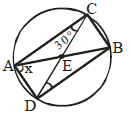Sol.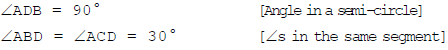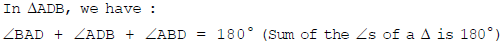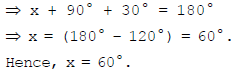Ex. If O is the centre of the circle, find the value of x in each of the following figures, giving reasons.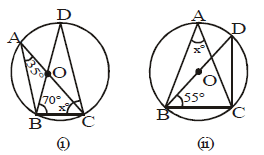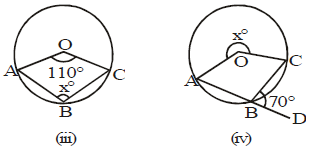Sol. We have:

(i) ∠BDC = ∠BAC = 35° [∠s in the same segment].

In ΔDBC, we have

∠DBC + ∠BCD + ∠BDC = 180° [Sum of the ∠s of a Δ is 180°]

⇒ 70° + x° + 35° = 180°

⇒ x° = (180° – 105°) = 75°.

Hence, x = 75°.

(ii) ∠BCD = 90°. [Angle in a semi-circle]

In ΔBCD, we have

∠BCD + ∠CDB + ∠DBC = 180° [Sum of the ∠s of a Δ is 180°]

⇒ 90° + ∠CDB + 55° = 180°

⇒ ∠CDB = (180° – 145°) = 35°.

∴ x = ∠BAC = ∠CDB = 35°. [∠s in the same segment]

Hence, x = 35°.

(iii) Reflex ∠AOC = (360 – 110)° = 250°.

Major arc CA subtends reflex ⇒AOC at the centre and ⇒ABC at a point B on the remaining part of the circle.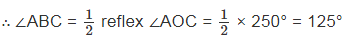Hence, x = 125°.

(iv) Since ABD is a straight line, we have

∠ABC + ∠CBD = 180°

⇒ ∠ABC + 70° = 180°

⇒ ∠ABC = (180° – 70°) = 110°.

Reflex ∠AOC = 2 ∠ABC = (2 × 110°) = 220°.

[Major arc CA subtends reflex ∠AOC at the centre and ABC at a point B on remaining part of the circle.]

∴ x = 220°.

Ex.20 In the figure, AB is a diameter of a circle with centre O and CD ║ BA.

If ∠BAC = 20°, find the values of
(i) ∠BOC

(ii) ∠DOC

(iii) ∠DAC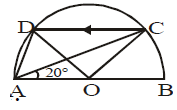Sol. (i) Arc BC subtends ∠BOC at the centre and ∠BAC at the circumference.

∴ ∠BOC = 2∠BAC = (2 × 20°) = 40°. [∵ Angle at the centre is double the angle at circumference]

(ii) ∠OCD = ∠BOC = 40° [Alt. interior ∠s as CD ║ BA]

Now, OC = OD [Radii of the same circle]

⇒ ∠ODC = ∠OCD = 40°.

In ∠OCD, we have

∠DOC + ∠OCD + ∠ODC = 180° [Sum of the ∠s of a Δ is 180°]

⇒ ∠DOC + 40° + 40° = 180°

⇒ ∠DOC = (180° – 80°) = 100°.

(iii) ∠DAC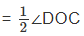=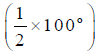= 50°.        [Angle at the centre is double the ∠ at circumference]

(iv) ∠ACD = ∠CAB = 20° [Alt. Int. ∠s, as CD ║ BA]

In ΔACD, we have

∠ADC + ∠ACD + ∠DAC = 180° [Sum of the ∠s of a Δ is 180°]

⇒ ∠ADC + 20° + 50° = 180°

⇒ ∠ADC = (180° – 70°) = 110°.

Hence, ∠BOC = 40°, ∠DOC = 100°, ∠DAC = 50° and ∠ACD = 110°.

The document Circle Passing through 3 points, Cyclic Quadrilateral and Related Theorems, Class 9, Mathematics | Extra Documents & Tests for Class 9 is a part of the Class 9 Course Extra Documents & Tests for Class 9.
All you need of Class 9 at this link: Class 9

## Extra Documents & Tests for Class 9

1 videos|228 docs|21 tests

## FAQs on Circle Passing through 3 points, Cyclic Quadrilateral and Related Theorems, Class 9, Mathematics - Extra Documents & Tests for Class 9

 1. What is a cyclic quadrilateral?Ans. A cyclic quadrilateral is a quadrilateral that can be inscribed in a circle, where all four vertices of the quadrilateral lie on the circumference of the circle.
 2. How many points are required to uniquely determine a circle passing through them?Ans. Three non-collinear points are required to uniquely determine a circle passing through them. These three points must not lie on a straight line.
 3. How can we prove that a quadrilateral is cyclic?Ans. To prove that a quadrilateral is cyclic, we need to show that the sum of opposite angles is equal to 180 degrees. If the sum of opposite angles of a quadrilateral is 180 degrees, then the quadrilateral is cyclic.
 4. Can a triangle be a cyclic quadrilateral?Ans. No, a triangle cannot be a cyclic quadrilateral. A cyclic quadrilateral must have four sides and four angles, whereas a triangle has only three sides and three angles. A triangle can be inscribed in a circle, but it cannot be considered a cyclic quadrilateral.
 5. What are some properties of a cyclic quadrilateral?Ans. Some properties of a cyclic quadrilateral are: - The opposite angles of a cyclic quadrilateral are supplementary, i.e., their sum is 180 degrees. - The exterior angle formed by extending one side of a cyclic quadrilateral is equal to the interior opposite angle. - The diagonals of a cyclic quadrilateral intersect at a point called the circumcenter, which is the center of the circle passing through all four vertices.

## Extra Documents & Tests for Class 9

1 videos|228 docs|21 testsExplore Courses for Class 9 examSignup to see your scores go up within 7 days! Learn & Practice with 1000+ FREE Notes, Videos & Tests.
10M+ students study on EduRev
Track your progress, build streaks, highlight & save important lessons and more!
Related Searches

,

,

,

,

,

,

,

,

,

,

,

,

,

,

,

,

,

,

,

,

,

,

,

,

,

,

,

,

,

,

;